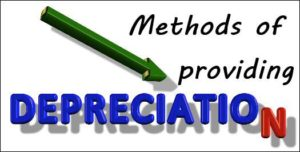# Methods of Calculating Depreciation Amount: SLM, Annuity Method

The compilation of these Depreciation, Provision and Reserves Notes makes students exam preparation simpler and organised.

## Methods of Calculating Depreciation Amount

Depreciation is a simple and interesting concept to study in accountancy. It holds the vital answer to the amount of expense which is charged to the profit and loss account during a period. The amount of depreciation, like other expenses, impacts the amount of profit earned or loss incurred during a year. Therefore, it is important to study the different methods, such as SLM, annuity method, etc. Let us study these methods.

### The Methods of Charging DepreciationStraight Line Method (SLM)
Straight-line depreciation is the most commonly used depreciation method in accounts and is used mainly owing to its simplicity. For the application of the straight-line method, a firm charges an equal amount of the asset’s cost to each accounting period. The straight-line formula that is used to calculate depreciation expense is as follows:

Asset’s historical cost – the asset’s estimated salvage value / the asset’s useful life

Units of Production
The units of production depreciation method allocate an equal amount of expense to each unit produced or service rendered by the asset. This method is usually applied to assets used in the production line. The formula to calculate depreciation expense involves the following steps:

Determine the depreciation per unit:
(Asset’s historical cost – estimated salvage value) / estimated total units of production during the asset’s useful life

Determine the expense for the accounting period:
(Depreciation per unit X number of units produced in the period)

Sum of Year’s Digits
Sum of years’ digits is a depreciation method that results in a more accelerated write-off of the asset than the straight-line method, but less accelerated than that of the double-declining balance method. Under this method, annual depreciation is determined by multiplying the depreciable cost by a series of fractions based on the sum of the asset’s useful life digits. The sum of the digits can be determined by using the formula:
(n2 + n)/2, where n is equal to the useful life of the asset

Double Declining Balance
The double-declining balance method is a type of accelerated depreciation method that calculates a higher depreciation charge in the first year of an asset’s life and gradually decreases depreciation expense in subsequent years. This method is used if the organization wants to increase the expenses for the year, to reduce tax liability.

Annuity Method
Annuity depreciation methods are usually not based on time, but on a level of Annuity. This could be miles driven for a vehicle, or a cycle count for a machine. When the asset is acquired, its life is estimated in terms of the level of activity or annuity. Assume a vehicle above is estimated to go 50,000 miles in its lifetime. The per-mile depreciation rate is calculated as follows:

(INR 17,000 cost – INR 2,000 salvage) / 50,000 miles = INR 0.30 per mile

Each year, the depreciation expense is then calculated by multiplying the number of miles driven by the per-mile depreciation rate.

Example:

Question:
Discuss the group depreciation method.